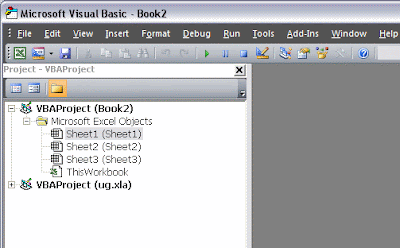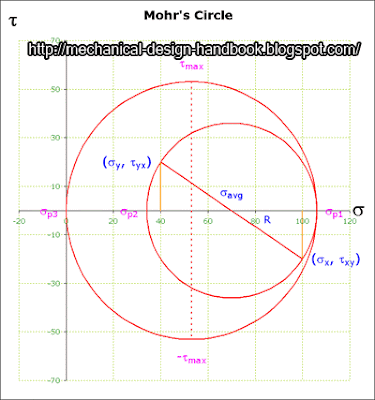## Posts

Showing posts from 2008

### Improve math skills of your kids - Learn step-by-step arithmetic from Math games

Math: Unknown - Step-by-step math calculation game for iOS.

Math: Unknown is much more than a math game. It is a step-by-step math calculation game which will teach users how to calculate in the correct order rather than just asking only the final calculated results.

The app consists of four basic arithmetic operations which are addition, subtraction, multiplication and division. In order to get started, users who are new to arithmetic can learn from animated calculation guides showing step-by-step procedures of solving each type of operation. It is also helpful for experienced users as a quick reference.

Generally, addition and subtraction may be difficult for users who just start learning math especially when questions require carrying or borrowing (also called regrouping). The app helps users to visualize the process of carrying and borrowing in the way it will be done on paper. Once users understand how these operations work, they are ready to learn multiplication and division.

For most students, division is considered as the most difficult arithmetic operation to solve. It is a common area of struggle since it requires prior knowledge of both multiplication and subtraction. To help users understand division, the app uses long division to teach all calculation procedures. Relevant multiplication table will be shown beside the question. Users will have to pick a number from the table which go into the dividend. Multiplication of selected number and divisor is automatically calculated, but the users have to do subtraction and drop down the next digit themselves. Learning whole calculation processes will make them master it in no time.

Math: Unknown is a helpful app for students who seriously want to improve arithmetic calculation skills.

### Dynamic Simulation using UG NX4 Motion Simulation ModuleWhich software are you using in your mechanical design project?

I have been using Solid Edge 3D CAD software for 1-2 years for my mechanical design projects in the past. But now, according to the standardization in my design organization, I have to switch to use Unigraphics software instead, or we call it in short as "UG NX4" (the latest version is NX6).

To be honest, at first I still like Solid Edge because I think I can make modeling and assembly in Solid Edge faster and easier than in UG. But one of the good thing for UG is Motion Simulation module that help me simulate the movement of mechanical assemblies before releasing for manufacturing. I know that Solid Edge also has this kind of simulation module, but I didn't have a chance to use (no license). So in this post, I would like to share how easy to set up motion simulation in UG NX4.

I would like to see how the cylinder moves when it is pushed by the rotating lever. So …

### Motion Simulation in Solid Edge using sketches together with Microsoft Excel VBA Programming Part IIIIn previous example, I showed how to control variables in Solid Edge sketch using direct link to excel spreadsheet. In this example, I am going to show how to use VBA (Visual Basic for Application) in excel to control the variables in Solid Edge sketch.

1) Create new file in Microsoft Excel

2) Press Alt+F11 to enter Microsoft Visual Basic windows as shown below

3) Right click in Project Explorer at new file name (in this example, new excel file name = Book2) > Insert > Module

4) To make Solid Edge type libraries available to the Visual Basic Object Browser, click References on the Project menu. On the References dialog box, select the Solid Edge libraries you want to access.

All variable automation is accessed through the Variables collection and Variable objects. The Variables collection serves two purposes: it allows you to create and access variable objects, and it allows you to work with dimensions as variables.

Note: When debugging programs that interact with the Variable Table, …

### Motion Simulation in Solid Edge using sketches together with Microsoft Excel VBA Programming Part IITo link solid edge variables to Microsoft excel, we can do by enter formula in the variables table or use VBA code.

In this post, I would like to show how easy to make it using variables table.You can use the Variable Table to define and edit functional relationships between the dimensions of a design in a familiar spreadsheet format. The Variable Table is accessed with the Variables command on the Tools menu. There are two types of variables: dimensions displayed in the design and variables you create directly in the Variable Table. Dimension variables directly control elements of a design. Variables that you create cannot directly control the design. A user variable must be set equal to a value or mathematical expression; for example, PI = 3.14159. Variables can control dimensional relationships by setting the dimensional relationship equal to the variable.Let's begin...

1) Open Solid Edge and make a sketch as shown below.

Mechanism in this example consists of cam, lever, p…

### Motion Simulation in Solid Edge using sketches together with Microsoft Excel VBA Programming Part I

Solid Edge has a very useful application called "Motion" which allows a user to simulate the movement of mechanical parts. This enables the machine designers to test and make sure that the movement of their mechanism is the same as they expect before manufacturing parts. This helps save a lot of money.

However, instead of using "Motion" application, I would like to show you how to simulate the motion of mechanical parts using a sketch in Solid Edge together with Microsoft Excel.

Why?

Most of the time, I started my mechanical design with the concept and layout using sketches. If we could simulate the motion of mechanical parts in a sketch, it would help me a lot.

I got this idea when I was playing with sketches and constraints in Solid Edge. Solid Edge allows us to change the dimensions easily by just rotating the mouse wheel.

Watch the following video to see how easy to make the motion in sketch.

So what I have to do is just to have Microsoft Excel VBA changes the …

### Microsoft Excel: Goal Seek Technique for solving mechanical engineering design's problemsProblems in mechanical design often require the solution of nonlinear equation such as
3x3 - 20x2 + 1000x + 12000 = 0
These problems are sometimes stated in the form, find the zeros or roots of the function
f(x) = 3x3 - 20x2 + 1000x + 12000 = 0
that is, find the values of x that make f(x) = 0. Therefore, they are sometimes called root solving problems. The equation shown above is a typical equation encountered in the problem of finding the maximum stress at a point. While such equations can be solved by trial and error (simply guessing value until the left-hand side of the equation is nearly zero), there exist simple, systematic ways to solve such problems. Two such methods - the Method of False Position and Newton-Raphson method.
The Method of False Position is a systematic method of narrowing down the region in which the root exists. And another one is the Newton-Raphson method of root solving. It is more sophisticate than the Method False Position. It uses the slope of the functi…

### Microsoft Excel VBA - Mohr's Circle for Plane StressThe German engineer Otto Mohr (1835-1918) developed a useful pictorial or graphical interpretation of the equations for finding the principal stresses and the maximum shearing stress at a point in a stressed member. This method, commonly called Mohr's circle, involves the construction of a circle in such a manner that the coordinates of each point on the circle represent the normal and shearing stresses on one plane through the stressed point, and the angular position of the radius to the point gives the orientation of the plane.

Normal stresses are plotted as horizontal coordinates, with tensile stresses (positive) plotted to the right of the origin and compressive stresses (negative) plotted to the left. Shearing stresses are plotted as vertical coordinates, with those tending to produce a clockwise rotation of the stress element plotted above the s axis, and those tending to produce a counterclockwise rotation of the stress element plotted below the s axis.

The results obtaine…

### Motion Simulation with Microsoft ExcelMotion Simulation with Microsoft Excel
Mechanical design usually deals with movement of several parts. There are a lot of softwares that help simulate the movement of concerned parts of machines such as UG, Solid Edge, Solid Work, etc.

But how do you make simulation without those softwares?

I use Microsoft Excel to do the simulation of my timing diagram. Watch the video.

Actually it is nothing but motion simulation using graphing method in Microsoft Excel. It cannot simulate all of your complex movements, but it helps you get the feeling of how your machine will behave according to your timing diagram.

This technique uses VBA (Visual Basic for Application) to control the movements. What you have to do is to prepare the excel spreadsheet with the table defining the sketch of your machine parts to be used in simulation. You have to set the formula in excel spreadsheet to manipulate the location (x,y) and rotation (angle). After proper settings, just let VBA code, which is embedded in Mi…

### Timing BeltsTiming belts solve the slip problems of flat, O ring, and V belts by using
a flexible tooth, molded to a belt that has tension members built in. The
teeth are flexible allowing the load to be spread out over all the teeth in
contact with the pulley. Timing belts are part of a larger category of
power transmission devices called synchronous drives. These belt or
cable-based drives have the distinct advantage of not slipping, hence the
name synchronous. Synchronous or positive drive also means these belts
can even be used in wet conditions, provided the pulleys are stainless
steel or plastic to resist corrosion.

Timing belts come in several types, depending on their tooth profile
and manufacturing method. The most common timing belt has a trapezoidal
shaped tooth. This shape has been the standard for many years,
but it does have drawbacks. As each tooth comes in contact with the mating
teeth on a pulley, the tooth tends to be deflected by the cantilever
force, deforming the belt’s teeth so that only …